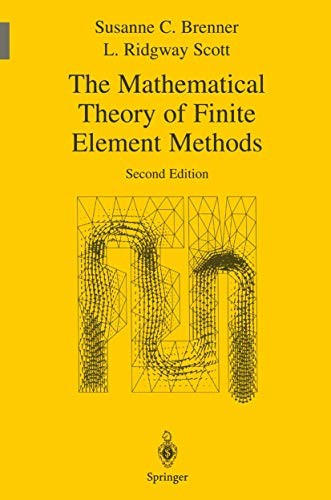# The Mathematical Theory of Finite Element Methods by Susanne C. Brenner

In Stock
£24.99
Develops the basic mathematical theory of the finite element method. This book is useful to mathematicians as well as engineers and physical scientists. It is useful for a course that provides an introduction to basic functional analysis, approximation theory, and numerical analysis.
Only 1 left

## The Mathematical Theory of Finite Element Methods Summary

### The Mathematical Theory of Finite Element Methods by Susanne C. Brenner

This book develops the basic mathematical theory of the finite element method, the most widely used technique for engineering design and analysis. It formalizes basic tools that are commonly used by researchers in the field but not previously published. The book will be useful to mathematicians as well as engineers and physical scientists. It can be used for a course that provides an introduction to basic functional analysis, approximation theory, and numerical analysis, while building upon and applying basic techniques of real variable theory. Different course paths can be chosen, allowing the book to be used for courses designed for students with different interests. For example, courses can emphasize physical applications, or algorithmic efficiency and code development issues, or the more difficult convergence theorems of the subject. This new edition is substantially updated with additional exercises throughout and new chapters on Additive Schwarz Preconditioners and Adaptive Meshes. Review of earlier edition: "This book represents an important contribution to the mathematical literature of finite elements. It is both a well-done text and a good reference." - "Mathematical Reviews, 1995".

### Why buy from World of BooksOur excellent value books literally don't cost the earthFree delivery in the UKEvery used book bought is one saved from landfill

## The Mathematical Theory of Finite Element Methods Reviews

From the reviews of the second edition: S.C. Brenner and L.R. Scott The Mathematical Theory of Finite Element Methods "[This is] a well-written book. A great deal of material is covered, and students who have taken the trouble to master at least some of the advanced material in the later chapters would be well placed to embark on research in the area. The book would work even better as a course text if computational and programming aspects of finite elements were to be integrated into the course work, or if a course on computational aspects of finite elements were offered in tandem."??? ZENTRALBLATT MATH "The authors have continued ??? the second edition, adding chapters on additive Schwarz preconditioners with applications to domain decomposition methods, and on a posteriori estimators and adaptivity. For researchers in finite elements and graduate students ??? this book is a valuable source, and provides an accessible route to the journal literature. ??? In summary, then, this is an excellent ??? introduction to key mathematical topics in the finite element method, and at the same time a valuable reference and source for workers in this area." (Batamanathan D. Reddy, Zentralblatt MATH, Vol. 1012, 2003) "This book is devoted to the mathematical theory of finite element method and is the second edition of the book from 1994. ??? The book can be used as a basis for graduate-level courses for students in applied mathematics, physics, engineering sciences and other fields ??? . The numerous and interesting exercises round offand complete each chapter. ??? The book can be highly recommended to everyone who is teaching or researching in the field of numerical solution of partial differential equations." (I. P. Gavrilyuk, Zeitschrift f??r Analysis und ihre Anwendungen, Vol. 22 (1), 2003)

Preface(2nd ed.).- Preface(1st ed.).- Basic Concepts.- Sobolev Spaces.- Variational Formulation of Elliptic Boundary Value Problems.- The Construction of a Finite Element of Space.- Polynomial Approximation Theory in Sobolev Spaces.- n-Dimensional Variational Problems.- Finite Element Multigrid Methods.- Additive Schwarz Preconditioners.- Max-norm Estimates.- Adaptive Meshes.- Variational Crimes.- Applications to Planar Elasticity.- Mixed Methods.- Iterative Techniques for Mixed Methods.- Applications of Operator-Interpolation Theory.- References.- Index.

GOR010298824
The Mathematical Theory of Finite Element Methods by Susanne C. Brenner
Susanne C. Brenner
Texts in Applied Mathematics
Used - Very Good
Hardback
Springer-Verlag New York Inc.
2002-04-01
388
0387954511
9780387954516
N/A
Book picture is for illustrative purposes only, actual binding, cover or edition may vary.
This is a used book - there is no escaping the fact it has been read by someone else and it will show signs of wear and previous use. Overall we expect it to be in very good condition, but if you are not entirely satisfied please get in touch with us.